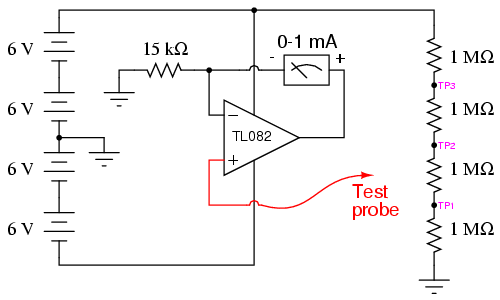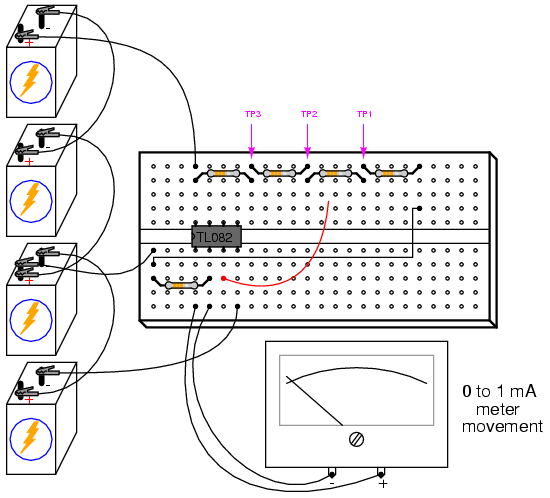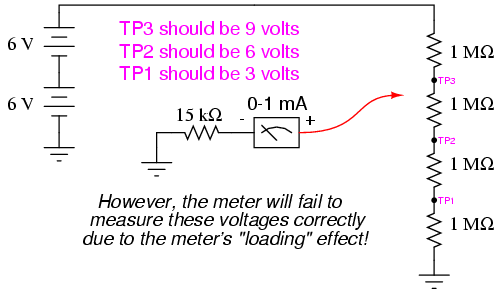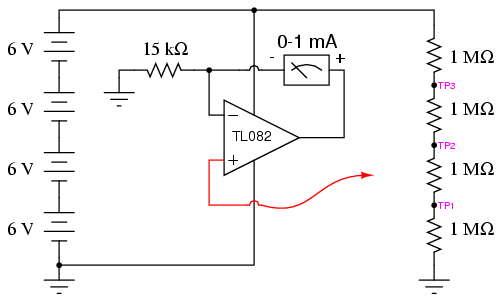» Experiment » Calculator/Converters » Radio » Newsletter » Associations and Societies » Component Manufacturers

 Electronics Symentics A B C D E F G H I J K L M N O P Q R S T U V W X Y Z

## High-impedance voltmeter

PARTS AND MATERIALS

• Operational amplifier, model TL082 recommended (Radio Shack catalog # 276-1715)

• Operational amplifier, model LM1458 recommended (Radio Shack catalog # 276-038)

• Four 6 volt batteries

• One meter movement, 1 mA full-scale deflection (Radio Shack catalog #22-410)

• 15 kΩ precision resistor

• Four 1 MΩ resistors

The 1 mA meter movement sold by Radio Shack is advertised as a 0-15 VDC meter, but is actually a 1 mA movement sold with a 15 kΩ +/- 1% tolerance multiplier resistor. If you get this Radio Shack meter movement, you can use the included 15 kΩ resistor for the resistor specified in the parts list.

This meter experiment is based on a JFET-input op-amp such as the TL082. The other op-amp (model 1458) is used in this experiment to demonstrate the absence of latch-up: a problem inherent to the TL082.

You don't need 1 MΩ resistors, exactly. Any very high resistance resistors will suffice.

CROSS-REFERENCES

Lessons In Electric Circuits, Volume 3, chapter 8: "Operational Amplifiers"

LEARNING OBJECTIVES

• How to make a high-impedance voltmeter using an op-amp

• What op-amp "latch-up" is and how to avoid it

SCHEMATIC DIAGRAMILLUSTRATIONINSTRUCTIONS

An ideal voltmeter has infinite input impedance, meaning that it draws zero current from the circuit under test. This way, there will be no "impact" on the circuit as the voltage is being measured. The more current a voltmeter draws from the circuit under test, the more the measured voltage will "sag" under the loading effect of the meter, like a tire-pressure gauge releasing air out of the tire being measured: the more air released from the tire, the more the tire's pressure will be impacted in the act of measurement. This loading is more pronounced on circuits of high resistance, like the voltage divider made of 1 MΩ resistors, shown in the schematic diagram.

If you were to build a simple 0-15 volt range voltmeter by connecting the 1 mA meter movement in series with the 15 kΩ precision resistor, and try to use this voltmeter to measure the voltages at TP1, TP2, or TP3 (with respect to ground), you'd encounter severe measurement errors induced by meter "impact:"Try using the meter movement and 15 kΩ resistor as shown to measure these three voltages. Does the meter read falsely high or falsely low? Why do you think this is?

If we were to increase the meter's input impedance, we would diminish its current draw or "load" on the circuit under test and consequently improve its measurement accuracy. An op-amp with high-impedance inputs (using a JFET transistor input stage rather than a BJT input stage) works well for this application.

Note that the meter movement is part of the op-amp's feedback loop from output to inverting input. This circuit drives the meter movement with a current proportional to the voltage impressed at the noninverting (+) input, the requisite current supplied directly from the batteries through the op-amp's power supply pins, not from the circuit under test through the test probe. The meter's range is set by the resistor connecting the inverting (-) input to ground.

Build the op-amp meter circuit as shown and re-take voltage measurements at TP1, TP2, and TP3. You should enjoy far better success this time, with the meter movement accurately measuring these voltages (approximately 3, 6, and 9 volts, respectively).

You may witness the extreme sensitivity of this voltmeter by touching the test probe with one hand and the most positive battery terminal with the other. Notice how you can drive the needle upward on the scale simply by measuring battery voltage through your body resistance: an impossible feat with the original, unamplified voltmeter circuit. If you touch the test probe to ground, the meter should read exactly 0 volts.

After you've proven this circuit to work, modify it by changing the power supply from dual to split. This entails removing the center-tap ground connection between the 2nd and 3rd batteries, and grounding the far negative battery terminal instead:This alteration in the power supply increases the voltages at TP1, TP2, and TP3 to 6, 12, and 18 volts, respectively. With a 15 kΩ range resistor and a 1 mA meter movement, measuring 18 volts will gently "peg" the meter, but you should be able to measure the 6 and 12 volt test points just fine.

Try touching the meter's test probe to ground. This should drive the meter needle to exactly 0 volts as before, but it will not! What is happening here is an op-amp phenomenon called latch-up: where the op-amp output drives to a positive voltage when the input common-mode voltage exceeds the allowable limit. In this case, as with many JFET-input op-amps, neither input should be allowed to come close to either power supply rail voltage. With a single supply, the op-amp's negative power rail is at ground potential (0 volts), so grounding the test probe brings the noninverting (+) input exactly to that rail voltage. This is bad for a JFET op-amp, and drives the output strongly positive, even though it doesn't seem like it should, based on how op-amps are supposed to function.

When the op-amp ran on a "dual" supply (+12/-12 volts, rather than a "single" +24 volt supply), the negative power supply rail was 12 volts away from ground (0 volts), so grounding the test probe didn't violate the op-amp's common-mode voltage limit. However, with the "single" +24 volt supply, we have a problem. Note that some op-amps do not "latch-up" the way the model TL082 does. You may replace the TL082 with an LM1458 op-amp, which is pin-for-pin compatible (no breadboard wiring changes needed). The model 1458 will not "latch-up" when the test probe is grounded, although you may still get incorrect meter readings with the measured voltage exactly equal to the negative power supply rail. As a general rule, you should always be sure the op-amp's power supply rail voltages exceed the expected input voltages.